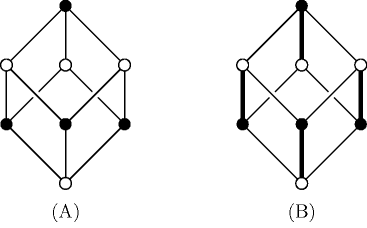### 3.7.33. Bipartite matching

##### Figure 3.7.8. (A) A bipartite graph and (B) one of its bipartite matchingDenotes that, for a given constraint, a bipartite matching algorithm can be used within its filtering algorithm. A bipartite matching is a subgraph that pairs every vertex of a bipartite graph with exactly one other vertex. A bipartite graph is a graph for which the set of vertices can be partitioned in two parts such that no two vertices in the same part are joined by an edge. Part (A) of Figure 3.7.8 shows a bipartite graph with a possible division of the vertices in black and white, while part (B) depicts with a thick line a bipartite matching of this graph.

A used generalisation so called degree-matching of a graph is a spanning sugraph where every vertex is associated with the bound degree of the matched edges.